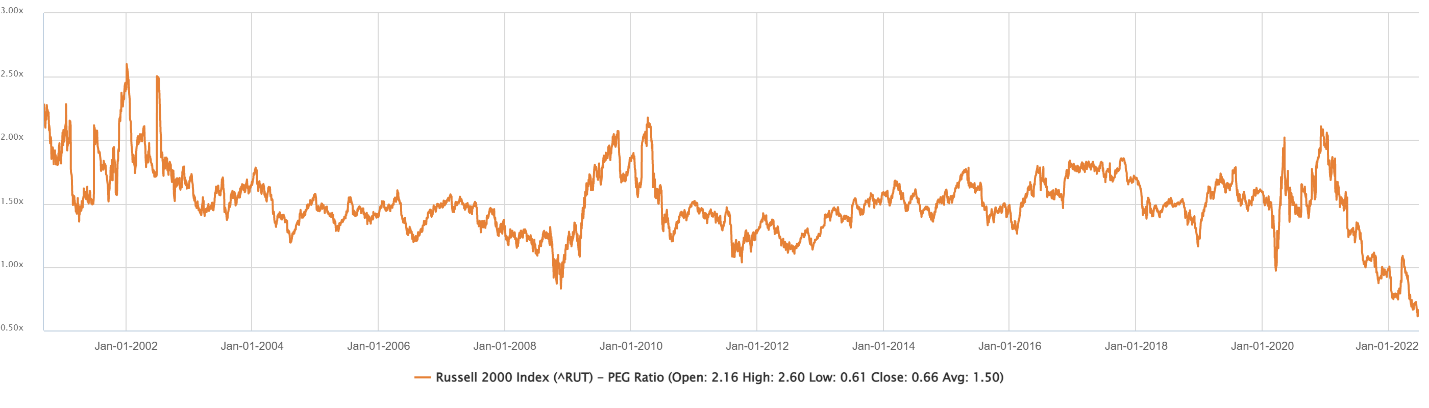My favorite fundamental indicator, the PEG ratio, shows small-cap stocks are a buy.

The indicator compares a stock’s price-to-earnings (P/E) ratio to its earnings growth rate. This simple calculation converts the popular P/E ratio to a meaningful data point.

The problem with the P/E ratio is that different stocks should have different ratios. But many investors use a static value to determine whether a stock is overvalued or undervalued.

A popular strategy is to buy a stock when the P/E ratio is below 15 or some other value. That rule ignores the fact that some stocks deserve higher valuations than others.

The PEG ratio addresses this problem.

## Small-Cap PEG Ratio Looks Promising

The indicator divides a stock’s P/E ratio by its growth rate in earnings. You can consider the stock a fair value when the PEG ratio equals one. The stock is undervalued if it’s below that level.

A PEG ratio above one indicates the stock is overvalued.

For example, if a tech company’s earnings grow at 50% a year, the PEG ratio is 0.8 for a P/E ratio of 40. For a slow-growth utility company, earnings growth of 8% and a P/E ratio of 12 result in a PEG ratio of 1.5.

Based on this, the utility stock is overvalued, while the tech stock is undervalued.

You can apply this calculation to indexes. The chart below shows the ratio for the Russell 2000, an index of small-cap stocks.

Russell 2000’s PEG Ratio Screams “Buy”Source: CapitalIQ.

The PEG ratio for the Russell 2000 is 0.66, a 20-year low. It’s also well below the 21-year average of 1.5. The ratio would need to increase by more than 220% to return to its average. This all means the Russell 2000 is undervalued.

Bottom line: As the Russell 2000 returns to its average, the value of the index should rise.

To participate in that recovery, investors could buy the iShares Russell 2000 ETF (NYSE: IWM). This is the most undervalued index right now and offers significant upside potential.

Michael Carr is the editor of True Options Masters, One Trade, Peak Velocity Trader and Precision Profits. He teaches technical analysis and quantitative technical analysis at the New York Institute of Finance. Follow him on Twitter @MichaelCarrGuru.